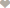# Nigeria Student Forum

Welcome, Guest: Join Nigeria Student Forum / Login / Trending Now / Recent

Stats: 17,629 members, 70,753 Topics, 15,563 Comments. Date: Jul 17 2019, 3:48 am

## QUESTION: If SinΦ= A/b , Then Where Φ Is Acute Then CotΦ = ? [Solved]

Nigeria Student Forum / Questions / Mathematics / If SinΦ= A/b , Then Where Φ Is Acute Then CotΦ = ?

(Go Down)
Answered: If SinΦ= A/b , Then Where Φ Is Acute Then CotΦ = ?8:36pm on January 11
Jonathan Irhodia

100Level, Nigeria.
Recall sinΦ = 1/cotΦ
if sinΦ= A/B
then A/B = 1/cotΦ
cotΦ = B/A2 UpVotes

Full Name:
Email: